# Odometer

The odometer is driven by rotation of the wheel whose diameter is 65 cm. After how many rotations the wheel turns counter to next kilometer?

Correct result:

n =  489.7

#### Solution: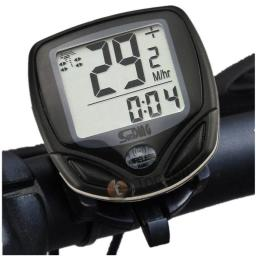We would be pleased if you find an error in the word problem, spelling mistakes, or inaccuracies and send it to us. Thank you!#### You need to know the following knowledge to solve this word math problem:

We encourage you to watch this tutorial video on this math problem:

## Next similar math problems:

• Bicycle wheel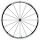Bicycle wheel diameter is 62 cm. How many times turns the bicycle on the road 1 km long?
• Pulley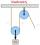On wheels with a diameter of 40 cm is fixed rope with the load. Calculate how far is load lifted when the wheel turns 7 times?
• Coal mine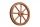The towing wheel has a diameter of 1.7 meters. How many meters does the elevator cage lower when the wheel turns 32 times?
• Wheel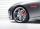How many times turns the wheel of a passenger car in one second if car run at speed 100 km/h. Wheel diameter is d = 62 cm.
• WellRope with a bucket is fixed on the shaft with the wheel. The shaft has a diameter 50 cm. How many meters will drop bucket when the wheels turn 15 times?
• Wheel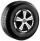What is the wheel diameter if on the 0.38 km track turns 128 times?
• Bicycle wheelAfter driving 157 m bicycle wheel rotates 100 times. What is the radius of the wheel in cm?
• Winch drum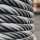Originally an empty winch drum with a diameter of 20 cm and a width of 30 cm on the rescue car, he started winding a rope with a thickness of 1 cm beautifully from edge to edge. The winch stopped after 80 turns. It remains to spin 3.54m of rope (without h
• Wheel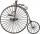Diameter of motocycle wheel is 52 cm. How many times rotates wheel on roand long 2 km?
• Round table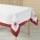A tablecloth should be sewn on a round table with a diameter of 78 cm, which should extend around the table by 10 cm. How many cms of ribbons need to be bought for edging?
• Bicycle wheels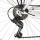Driving wheel of a bicycle has 54 teeth. The driven wheel has 22 teeth. After how many revolutions will meet the same teeth?
• Diameter to areaFind the area of a circle whose diameter is 26cm.Calculate the radius of the circle whose length is 107 cm larger than its diameter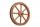A 1m diameter wheel rolled along a 100m long track. How many times did it turn?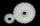Gearing consists of two wheels, one is 88 and second 56 teeth. How many times turns smaller wheel to fit the same teeth as in the beginning? How many times will turn biggest wheel?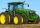Small tractor wheel with a diameter of 60 cm must be rotated 8 times to overcome some pathway. How many times must turn the big tractor wheel with a radius 60 cm to overcome the same distance?The diameter of the cylinder is 42 cm. How many times does the cylinder turn on a 66m long track?